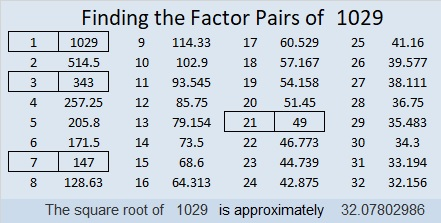# 1765 On This Memorial Day

### Today’s Puzzle:

This weekend I laid a bouquet of red and white flowers on my husband’s grave and decided to make a red rose Memorial Day puzzle for the blog as well. It is a mystery-level puzzle.

Write the number from 1 to 12 in both the first column and the top row so that those numbers are the factors of the given clues. There is only one solution.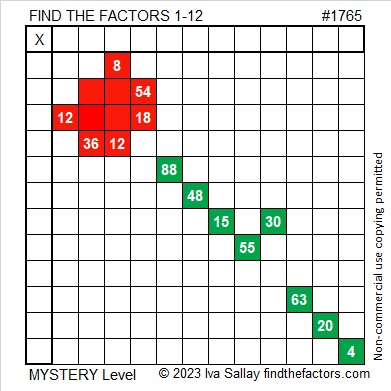### Factors of 1765:

• 1765 is a composite number.
• Prime factorization: 1765 = 5 × 353.
• 1765 has no exponents greater than 1 in its prime factorization, so √1765 cannot be simplified.
• The exponents in the prime factorization are 1 and 1. Adding one to each exponent and multiplying we get (1 + 1)(1 + 1) = 2 × 2 = 4. Therefore 1765 has exactly 4 factors.
• The factors of 1765 are outlined with their factor pair partners in the graphic below.### More About the Number 1765:

1765 is the sum of two squares in two different ways:
42² + 1² = 1765, and
33² + 26² = 1765.

1765 is the hypotenuse of FOUR Pythagorean triples:
84 1763 1765, calculated from 2(42)(1), 42² – 1², 42² + 1²,
413 1716 1765, calculated from 33² – 26², 2(33)(26), 33² + 26²,
1059-1412-1765, which is (3-4-5) times 353, and
1125-1360-1765, which is 5 times (225-272-353).

1765 is a digitally powerful number:
1⁴ + 7³ + 6⁴ + 5³ = 1765.

1765 is a palindrome in a couple of different bases:
It’s A5A base 13 because 10(13²) + 5(13) + 10(1) = 1765, and
it’s 1D1 base 36 because 1(36²) + 13(36) + 1(1) = 1765.

# 1745 How Thorny Is This Rose?

### Today’s Puzzle:

How thorny is this Valentine’s rose? That depends on if you use logic to solve it! It is a Level 5 puzzle, so some of the clues are meant to be tricky. Nevertheless, you can solve it! Just place all the numbers 1 to 10 in both the first column and the top row so that the given clues are the products of the numbers you write. There is only one solution.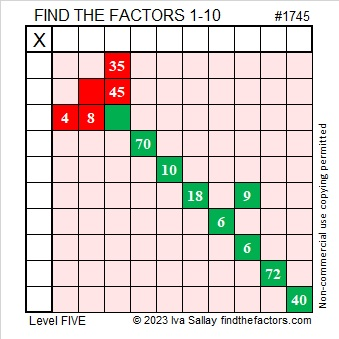Here’s the same puzzle minus the color: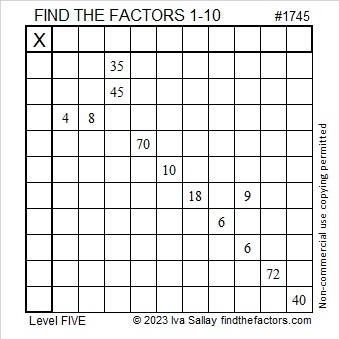### Factors of 1745:

• 1745 is a composite number.
• Prime factorization: 1745 = 5 × 349.
• 1745 has no exponents greater than 1 in its prime factorization, so √1745 cannot be simplified.
• The exponents in the prime factorization are 1 and 1. Adding one to each exponent and multiplying we get (1 + 1)(1 + 1) = 2 × 2 = 4. Therefore 1745 has exactly 4 factors.
• The factors of 1745 are outlined with their factor pair partners in the graphic below.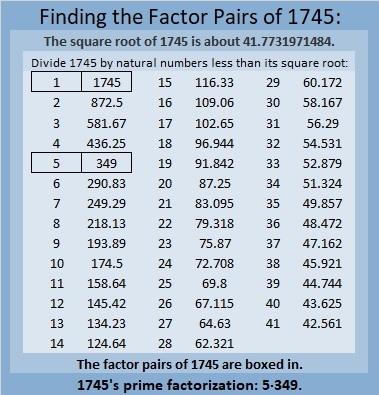### More About the Number 1745:

1745 is the difference of two squares in two different ways:
873² – 872² = 1745, and
177² – 172² = 1745.

1745 is also the sum of two squares in two different ways:
41² + 8² = 1745, and
31² + 28² = 1745.

1745 is the hypotenuse of FOUR Pythagorean triples:
177-1736-1745, calculated from 31² – 28², 2(31)(28), 31² + 28²,
656-1617-1745, calculated from 2(41)(8), 41² – 8², 41² + 8²,
900-1495-1745, which is 5 times (180-299-349)
1047-1396-1745, which is (3-4-5) times 349.

# 1029 A Rose for Your Valentine

A dozen roses can be pretty pricey around Valentine’s Day, but at least one website asserts that a single rose can make just as big a statement and just as big an impact. Today’s mystery level puzzle looks like a single rose. I hope you will enjoy its beauty even if its thorns are prickly.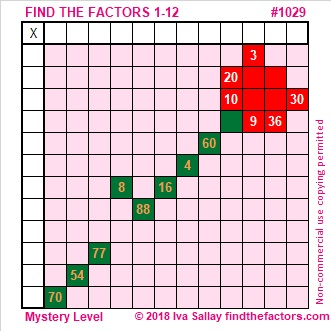Print the puzzles or type the solution in this excel file: 12 factors 1028-1034

Here are a few facts about the number 1029:

It’s easy to see that 1029 can be evenly divided by 3 because 1 + 0 + 2 + 9 = 12, a number divisible by 3.

It’s not quite as easy to tell that it can be evenly divided by 7:
It is because 102 – 2(9) = 102 – 18 = 84, a number divisible by 7.

I like the way 1029 looks when it is written in some other bases:
It’s 4433 in BASE 6 because 4(6³) + 4(6²) + 3(6¹) + 3(6⁰) = 4(216 + 36) + 3(6 + 1) = 1029,
3000 in BASE 7 because 3(7³) = 3(343) = 1029,
399 in BASE 17 because 3(17²) + 9(17) + 9(1) = 3(289 + 51 + 3) = 3(343) = 1029
333 in BASE 18 because 3(18² + 18 + 1) = 3(343) = 1029

• 1029 is a composite number.
• Prime factorization: 1029 = 3 × 7 × 7 × 7, which can be written 1029 = 3 × 7³
• The exponents in the prime factorization are 1 and 3. Adding one to each and multiplying we get (1 + 1)(3 + 1) = 2 × 4 = 8. Therefore 1029 has exactly 8 factors.
• Factors of 1029: 1, 3, 7, 21, 49, 147, 343, 1029
• Factor pairs: 1029 = 1 × 1029, 3 × 343, 7 × 147, or 21 × 49
• Taking the factor pair with the largest square number factor, we get √1029 = (√49)(√21) = 7√21 ≈ 32.07803